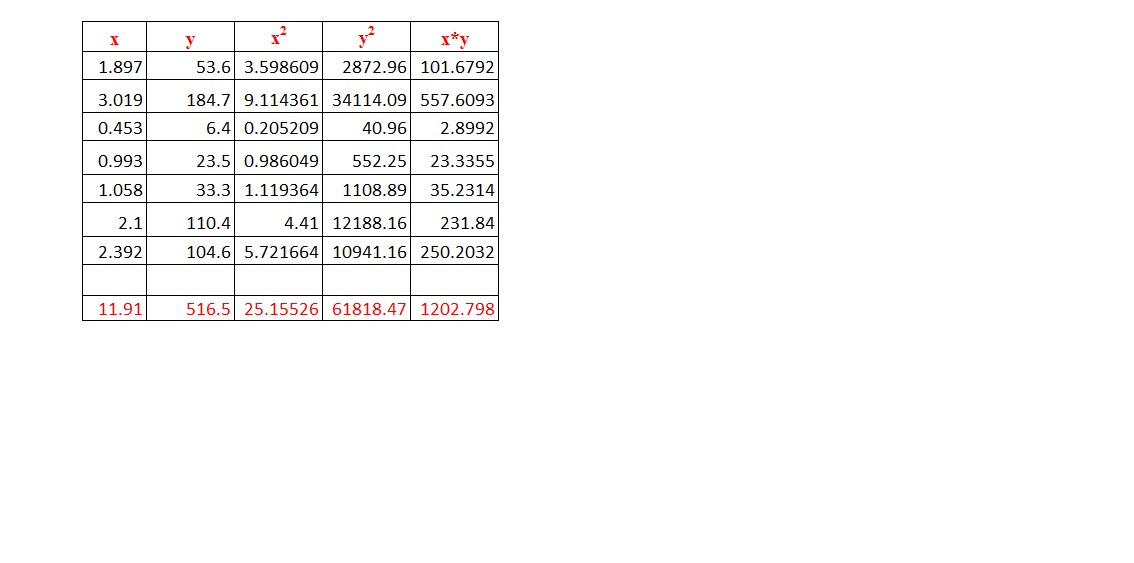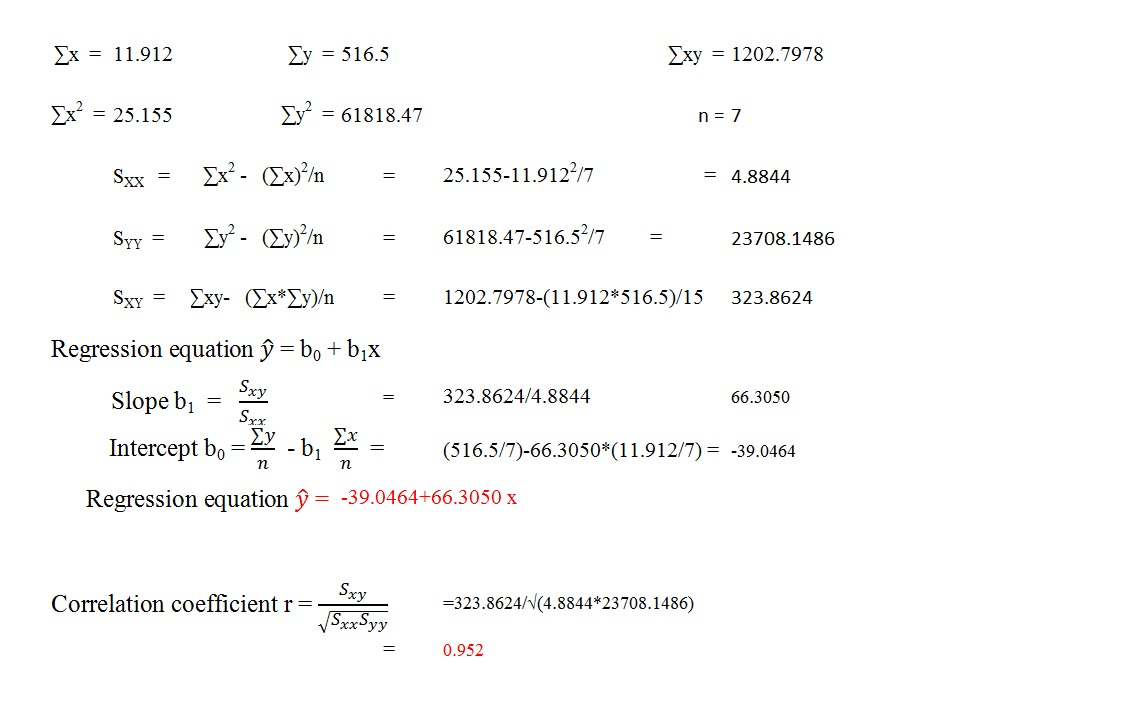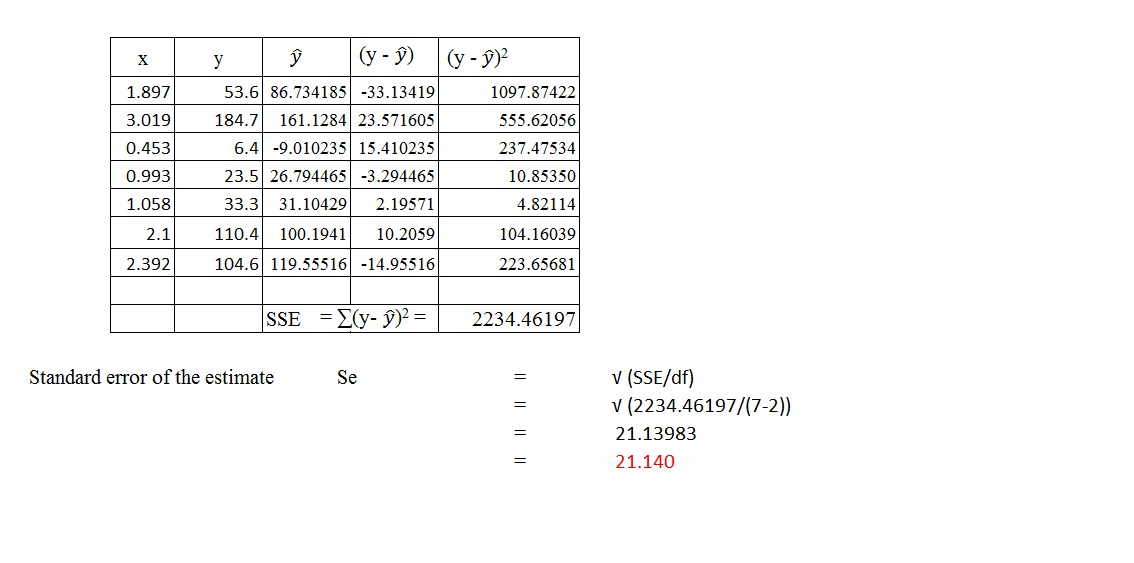##### Question

In: Statistics and Probability

# People in the aerospace industry believe the cost of a space project is a function of...

People in the aerospace industry believe the cost of a space project is a function of the mass of the major object being sent into space. Use the following data to develop a regression model to predict the cost of a space project by the mass of the space object. Determine r2 and se. Weight (tons) Cost ($millions) 1.897$ 53.6 3.019 184.7 0.453 6.4 0.993 23.5 1.058 33.3 2.100 110.4 2.392 104.6 *(Do not round the intermediate values. Round your answers to 4 decimal places.) **(Round the intermediate values to 4 decimal places. Round your answer to 3 decimal places.) ŷ = enter a number rounded to 4 decimal places * + enter a number rounded to 4 decimal places * x r2 = enter a number rounded to 3 decimal places ** se = enter a number rounded to 3 decimal places **

ŷ = enter a number rounded to 4 decimal places

* + enter a number rounded to 4 decimal places

* x
r2 = enter a number rounded to 3 decimal places  **
se = enter a number rounded to 3 decimal places  **

## Solutions

##### Expert Solution## Related Solutions

##### People in the aerospace industry believe the cost of a space project is a function of...
People in the aerospace industry believe the cost of a space project is a function of the mass of the major object being sent into space. Use the following data to develop a regression model to predict the cost of a space project by the mass of the space object. Determine r2 and se. Weight (tons) Cost ($millions) 1.897$ 53.6 3.019 $185.2 0.453$6.4 0.960 $23.5 1.058$33.5 2.100 $110.4 2.392$104.6 ŷ = ________ + __________ x...
##### People in the aerospace industry believe the cost of a space project is a function of...
People in the aerospace industry believe the cost of a space project is a function of the mass of the major object being sent into space. Use the following data to develop a regression model to predict the cost of a space project by the mass of the space object. Determine r2 and se. Weight (tons) Cost ($millions) 1.897$ 53.6 3.019 183.9 0.453 6.4 0.996 23.5 1.058 32.9 2.100 110.4 2.380 104.6 *(Do not round the intermediate values....
##### people in the aerospace industry believe the cost of a space project is a function of...
people in the aerospace industry believe the cost of a space project is a function of the mass of the major object being sent into space. use the following data to develop a regression model to predict the cost of a space project by the mass of the space object. determine r^2 and se. weight= 1.897, 3.019, 0.453, 0.970, 1.058, 2.100, 2.389. cost = 53.6, 183.9, 6.4, 23.5, 33.4, 110.4, 104.6. y= + x r^2= se=
##### People in the aerospace industry believe the cost of a space project is a function of...
People in the aerospace industry believe the cost of a space project is a function of the mass of the major object being sent into space. Use the following data to develop a regression model to predict the cost of a space project by the mass of the space object. Determine r2 and se. Weight (tons) Cost ($millions) 1.897$ 53.6 3.019 $184.1 0.453$6.4 0.996 $23.5 1.058$33.7 2.100 $110.4 2.400$104.6 *(Do not round the intermediate values....